2014-06-03

# A method to determine coefficients in Cauchy-Euler differential equations of order n

## Autores/as

• Fabio Hernando Castellanos Moreno
• Jaime Francisco Pantoja Benavides

## Palabras clave:

buckling, ODE, algorithm, Cauhy-Euler coefficients, reliability (es).

22

## PlumX

### Descargas

Los datos de descargas todavía no están disponibles.
A METHOD TO DETERMINE COEFFICIENTS IN CAUCHY-EULER DIFFERENTIAL EQUATIONS OF ORDER N

A METHOD TO DETERMINE COEFFICIENTS IN CAUCHY-EULER DIFFERENTIAL EQUATIONS OF ORDER N

submitted date: June 2013
accepted date: November 2013

Fabio Hernando Castellanos Moreno

B.Sc. In mathematics, Universidad Distrital Francisco José de Caldas (Colombia). B.Sc. In Electronic Engineering, Universidad Antonio Nariño (Colombia). M.Sc. In applied mathematics, Universidad EAFIT (Colombia). Current position: professor at Universidad Distrital Francisco José de Caldas and Universidad Central (Colombia), member of DIGITI investigation group, Universidad Distrital Francisco José de Caldas (Colombia). E-mail: fabiocastellanos@udistrital.edu.co

Jaime Francisco Pantoja Benavides

B. Sc. In mathematics, Universidad Distrital Francisco José de Caldas (Colombia). Specialist in statistics, Universidad Nacional de Colombia (Colombia). Current position: professor at Universidad Distrital Francisco José de Caldas Bogotá (Colombia). Current position: professor at Universidad Distrital Francisco José de Caldas Bogotá (Colombia), research professor of DIGITI investigation group Universidad Distrital Francisco José de Caldas (Colombia). E-mail: jfpantojab@udistrital.edu.co.

Abstract

This article presents a method that provides a simple way to find the constant coefficients required in the solution of a differential equation with Cauchy-Euler structure. Although this method is not fundamental in the solution of a differential equation of this type, it does propose an innovative and practical vision of differential equations of higher order with Cauchy structure in order to find the constant coefficients. Firstly, the article offers an introduction to differential equations and their usefulness (e.g. solid mechanics, buckling of columns); then, the structure of a Cauchy-Euler equation is presented followed by the proposed method, including mathematical proof, which guarantees the reliability of the method. Finally, conclusions are drawn regarding the usefulness of the method.

Key words

buckling, ODE, algorithm, Cauhy-Euler coefficients, reliability.

Introduction

One of the most important aspects of mathematics lies in the fact that this discipline of knowledge emerged from the moment in which the man had the ability to discern, motivated by the need to understand many situations his surrounding world. Whether mathematics allows to directly (or indirectly) solve such situations, it permits establishing models that represent a particular problem and, through a series of processes and operations, gives solutions to a mathematical model, which then becomes the answer the problem. A large number of these situations have been taken from the study of the basic sciences, especially from physics (e.g. solid mechanics, buckling of columns) [1-2], and most such solutions have used the type of mathematical modeling that leads to differential equations, which have been studied in different ways and with different approaches, either according to their difficulty or according to their properties and characteristics. Similarly, when applying these equations, it is important not to let oneself be overwhelmed by the beauty of mathematics and lose the focus on the features and properties of equations, forgetting their fundamental purpose; instead, one must try to focus on giving solutions to real-world situations; that is, in many aspects the main concern has been the “how”, forgetting the “why” and “what for”. This happens not only in the study of differential equations, but in mathematics in general, [3-5].

Now, the first studies involving differential equations, performed by J. Napier at the end of the 16th century, led to expressions which today are written as: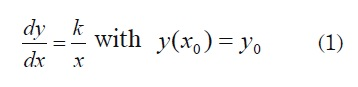This led Napier to define the concept of logarithm and implicitly use the method that later would be called separable variables. Later other scholars of knowledge, like Galileo used differential equations for the solution of movement with variable speed, e.g. free fall or the study of optical phenomena (as conducted by Descartes). Thus, many mathematical physicists made various contributions to the study of the differential equations, but as a way to solve particular situations. Newton in his theories about physics was someone that made strides to substantiate the very construction of classical mechanics. The same thing happened later with Euler, Leibniz, Ricatti, Clairaut, D’Alembert, the Bernoulli brothers and others. The work developed by Euler, starting in 1743, about the applications of ordinary and partial differential equations, in of particular linear ordinary differential equations with constant coefficients with substitutions y = ekx and y = uekx in the case of multiple roots, shows that the homogeneous linear equation with constant coefficients of order n is a linear combination of its n particular solutions; among other contributions of great importance to the solution of differential equations in general. After the middle of the 19th century the role played by the integral raised by Cauchy began to thrive. Cauchy made important contributions to the construction of the theory of differential equations, giving solution to the first order equations during the 1820s decade in the following way: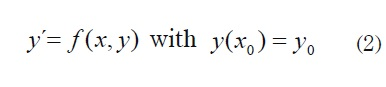and proof of the uniqueness of such solution, assuming the continuity of f and ∂f/∂y, and his generalization [ 6], .

1. Cauchy-Euler equation

The differential equation known as Euler- Cauchy, in its homogeneous form, is an equation of the form: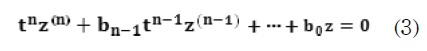Where it is satisfied that for each term, the coefficient of the n-th term, differentiated from the dependent variable is the n-th power of the independent variable. Now, the substitution t = eu reduces equation (3) to a linear equation of order n with constant coefficients and this result describes a Fundamental System of Solutions of the Cauchy equation .

It is known that if t = eu then Ln (t) = u

where dt/t = du and therefore 1/t = du/dt

Then, as in (3), each differentiation from z is made with respect to t, using rates of change, one may write: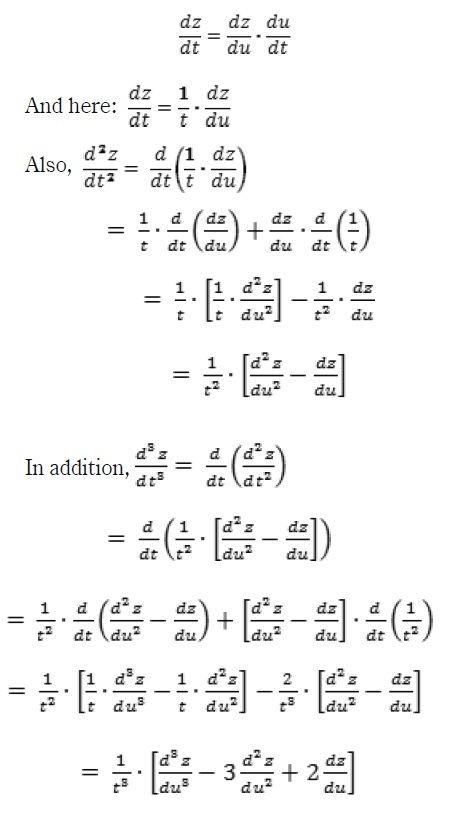That is to say,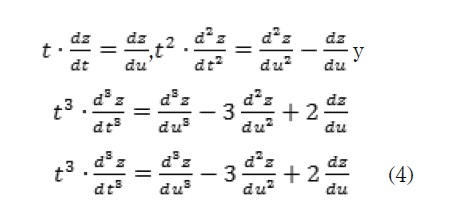2. Proposed method

Recently, in  be use the Mellin Transform for solve (1) of alternative way. However, In this paper, an analysis of the equivalences given in (4), for the products of the form tn * dnz / dtn leads to propose an iterative process that allows finding the real coefficients, real akj values of the resulting terms in the conversion process (3), to a homogeneous differential equation of order n with constant coefficients.

Such method or process is based on the fact that each term of equation (3) can be transformed into a differential expression with constant coefficients, through the following iterative equation: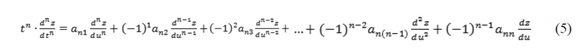Where: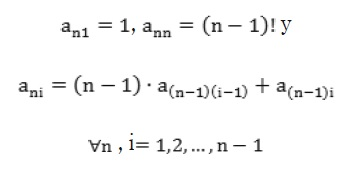Keep in mind that every term of the form tn * dnz / dtn becomes a differential expression of order n, but with n terms with constant coefficients. These coefficients are of the form akj where k indicates the order of expression tk * dkz / dtn and j indicates the term that represents the coefficient in the differential expression found.

The following is a demo, by induction, of the method proposed in (5):

Demonstration 1:

Suppose that the method holds for n = k; and if it holds for n = k + 1, assume that itWhere: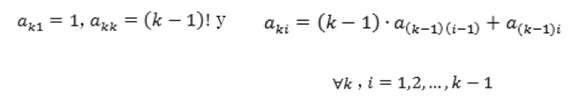it must be verified that the following also holds: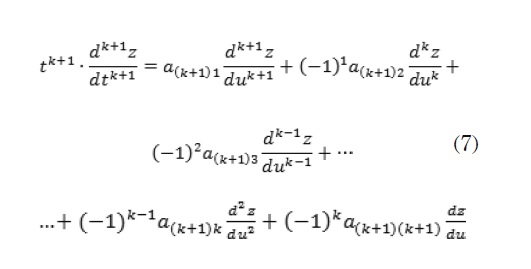Where: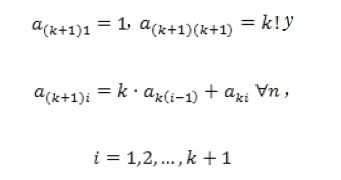Then: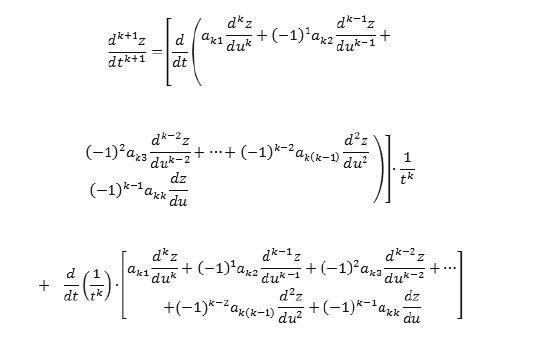Considering the conclusion given in (4), the following is obtained: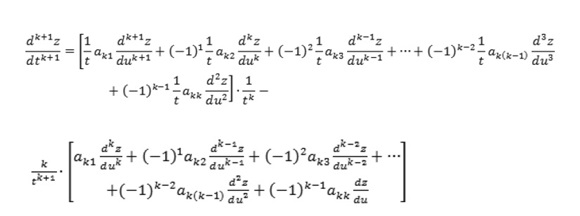Simplifying,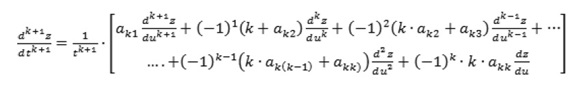As ak1= 1 we can make, ak1= a(k+1)1=1 and also akk = (k-1)!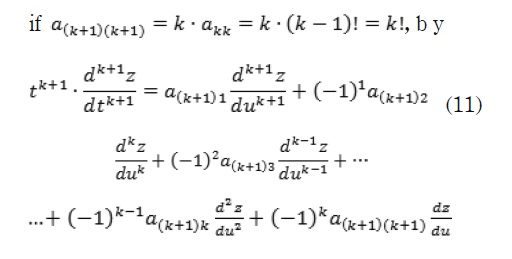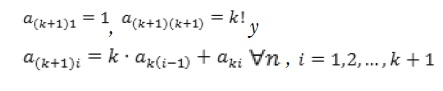This proves that the equation (5) is correct.

Then by substituting each of the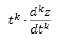in the equation (3), developed as shown in (5), the coefficients of the resulting expression are real constant, that means that they can be simplified to an expression of the form: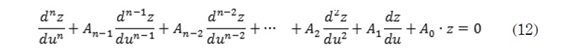which is a homogeneous differential equation of order n with constant coefficients. Now, if L(r) is the characteristic polynomial of (12) and its roots are r1, r2...rm whit m ≤ n, then a fundamental system of solutions to (12) is: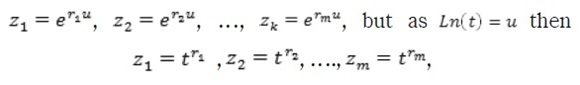Which would be a fundamental system of solutions to (3). Now, if some rk = α ± iβ is a complex root , then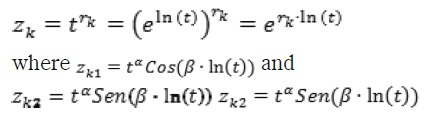On the other hand, if some root r has multiplicity k then these k solutions would be: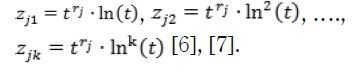To understand the use of this algorithm, for the case n = 2 in (3), the following equation holds: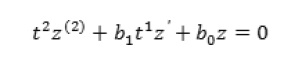and by t= eu one gets the equations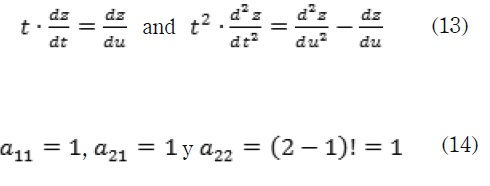Which perfectly matches (7), now substituting (8) in (5) for n = 3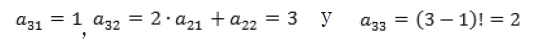from this point onwards, i.e. equation (5) with n=3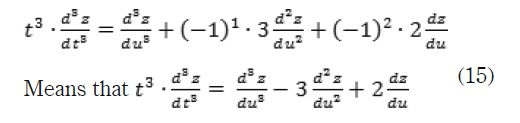Which is exactly the same equation found in (4). Now, repeating the same process for n = 4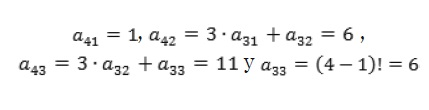Therefore,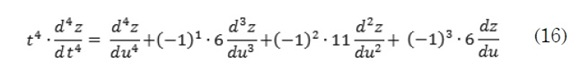We invite the reader to do the long and unusual process of differentiation of (9) and prove that it reaches the same result found in (16), which is presented in a very simple way with the method proposed here.

The following graphic 1, shows ways in which this method works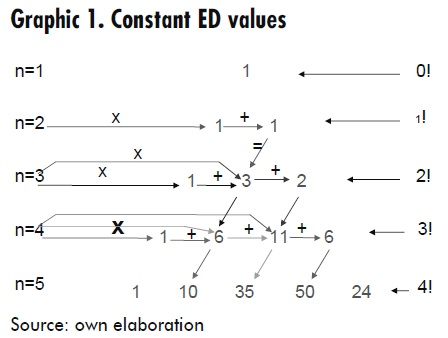3. Conclusions

The study and application of differential equations has allowed the development of many Sciences and in particular the advance of technology. Thus, for the academic community it is very important to have methods to solve such equations in a practical and fast manner so that developments and applications in technology can be widely implemented, e.g. solid mechanics and particularly buckling of columns in structures. The method presented in this article, is not a different process to the already-known for differential equations with Cauchy-Euler structure. Simply, the present paper sets a series of steps and alternate processes (new proposal) that allow obtaining the linear differential equation with constant coefficients, avoiding the long path of differentiation, whose last equation is known to be easily solved with auxiliary features equations and factoring elementary processes.

The presentation of new proposals that contribute to the development or simplification of the sciences, allows thinking that it is still possible to continue transforming science by making it simpler and easy-to-use through the use of technological resources and software tools, based on mathematics.

References

 E . Popov, “Introducción a la mecánica de los sólidos”. México D.F: Ed. Limusa, 1981.

 P. A. Lagace, “Unified Engineering”, 2009, disponible en: http://web.mit. edu/16.unified/www/SPRING/materials/ Lectures/M4.7-Unified09.pdf

 D.G. Zill, "Applications of modeling with differential equations", Cengage Learning Editores S.A. 2009, ninth edition, pp. 162-67.

 I. Ellsgoltz, "Differential equations and Variational calculation", Publishing House MIR Moscow, 1969, pp. 110-116..

 E . Blaksley, "Special methods for differential equations". Editorial Universitaria de Buenos Aires. 1969, pp. 5-7.

 M . Guzman, "Stability theory-ordinary differential equations and Control". Editorial Alhambra, S.A. Madrid 1975, pp. 18-24.

 T. Mynt-U and L. Debnath. Linear Partial Diferential Equations for Scientists and Engineers, Birkhäuser, Boston 2007, Boston-Basel-Berlin, pp. 1-12.

 J.R. González and L.F.Plaza G, "Solution of the equation Cauchy-Euler by the Mellin Transform" Scientia et Technica, año XV, Agosto de 2009